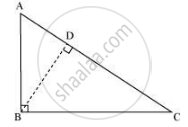# Prove that in a Right Angle Triangle, the Square of the Hypotenuse is Equal to the Sum of Squares of the Other Two Sides. - Mathematics

Sum

Prove that in a right angle triangle, the square of the hypotenuse is equal to the sum of squares of the other two sides.

#### Solution

“In a right triangle, the square of the hypotenuse is equal to the sum of the squares of the other two sides.”

Proof: Let ABC be a right triangle where ∠B = 90°.

It has to be proved that AC2 = AB2 + BC2

Construction: Draw BD ⊥ AC∠ADB = ∠ABC [Each is right angle]

Therefore, by AA similarity criterion, ΔADB ∼ ΔABC

("AD")/("AB") = ("AB")/("AC")   .....[Sides are proportional in similar triangles]

⇒ AD x AC = AB2  ...(1)

Similarly, it can be proved that ΔBDC ∼ ΔABC

("CD")/("BC") = ("BC")/("AC")

⇒ AC x CD = BC2  ...(2)

Adding equations (1) and (2), we obtain

AB2 + BC2 = AD × AC + AC × CD

⇒ AB2 + BC= AC (AD + CD)

⇒ AB2 + BC2 = AC × AC

⇒ AB2 + BC2 = AC2

This proves the Pythagoras Theorem.

Concept: Right-angled Triangles and Pythagoras Property
Is there an error in this question or solution?